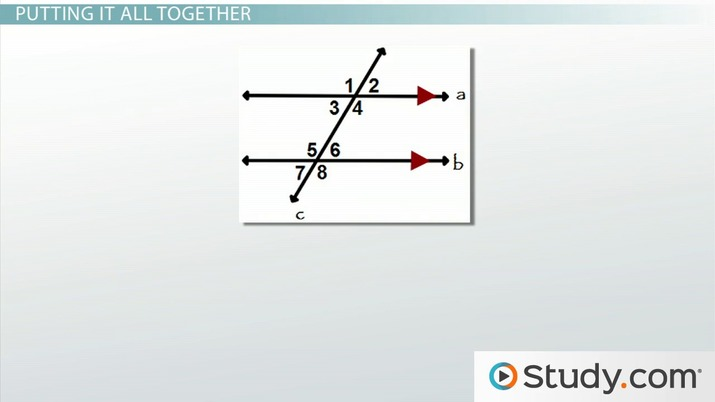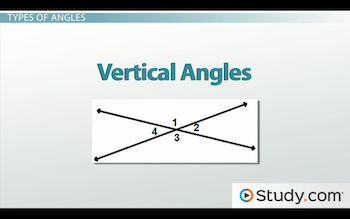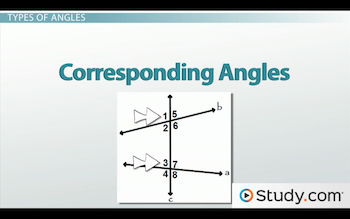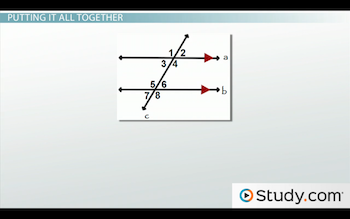# Types of Angles: Vertical, Corresponding, Alternate Interior & Others

Lesson Transcript
Instructor: DaQuita Hester

DaQuita has taught high school mathematics for six years and has a master's degree in secondary mathematics education.

Understanding angles and angle relationships allows for easier calculation of angle measurement. Learn how to define various types of angles, including vertical, corresponding, alternate interior angles, and how to calculate degree measurement using angle relationships. Updated: 08/25/2021

## Angles are Everywhere

Have you ever looked at a sliced pizza and noticed that the beginning of each pizza slice was the same size? Did you ever take the time to wonder why that was? Or, have you ever examined the lines in a parking lot? While you may have noticed all the lines that form the parking spaces, did you ever think about the angles that were formed?

Well, if you haven't before, I'm sure you're thinking of them now. In every pizza and in every parking lot, there are many different angles and angle relationships. In this lesson, we are going to learn about these relationships, ways to identify the relationships and examine the measures of these angles.An error occurred trying to load this video.

Try refreshing the page, or contact customer support.

Coming up next: Angles and Triangles: Practice Problems

### You're on a roll. Keep up the good work!

Replay
Your next lesson will play in 10 seconds
• 0:05 Introduction
• 0:43 Types of Angles
• 2:30 Determining Angle…
• 4:03 Angle Measures
• 4:53 Putting It All Together
• 9:24 Lesson Summary
Save Save

Want to watch this again later?

Timeline
Autoplay
Autoplay
Speed Speed

## Types of Angles

The first angle relationship that we will discuss is vertical angles. They are defined as a pair of nonadjacent angles formed by only two intersecting lines. They are known as 'Kissing Vs' and always have congruent measures. In the figure below, angles 1 and 3 are vertical, as well as angles 2 and 4.The second relationship is corresponding angles. They are considered to be in the same location at each point of intersection. For example, take a look at angles 1 and 3 below. They are both in the upper left corner. Another pair of corresponding angles is angles 6 and 8, which are both in the lower right corner.Next we have alternate interior angles. Located between the two intersected lines, these angles are on opposite sides of the transversal. Angles 2 and 7 above, as well as angles 3 and 6 are examples of alternate interior angles.

Similarly, we also have alternate exterior angles that are located outside of the two intersected lines and on opposite sides of the transversal. An example of this relationship would be angles 1 and 8, as well as angles 4 and 5.

The last angle relationship is consecutive interior angles. These angles are located on the same side of the transversal and inside of the two lines. In the diagram above, angles 2 and 3 are consecutive interior angles, and so are angles 6 and 7.

With the exception of vertical angles, all of these relationships can only be formed when two lines are intersected by a transversal.

## Determining Angle Relationships

With so many similarities, you may be wondering how to determine the relationship between angles formed by transversals and intersecting lines. All you have to do is ask yourself these three basic questions:

1. Are the angles in the same location at both points of intersection? This means that we want to know if they are both in the upper left, upper right, lower left, or lower right corners of the intersections. If you determine that they are in the same location, then these angles must be corresponding angles, and you are finished. If they are not corresponding angles, move on to questions 2 and 3.
2. Are the angles on the same side or opposite sides of the transversal? If they are on the same side, then the angles are considered consecutive. If they are on opposite sides, then the angles are considered alternate.
3. Are the angles inside or outside of the two intersected lines? If they are inside the two lines, then they will be classified as interior. If located outside the two lines, then the angles are considered to be exterior.

From here, we will combine our answers for questions 2 and 3 to determine the relationship between the angles.

Before we get into our examples, let's go ahead and discuss the relationship between the measures of these angles.

## Angle Measures

As mentioned earlier, a pair of vertical angles will always be congruent. No matter what the diagram looks like, each pair of vertical angles will always have the same measurement. But, the story is a little different when observing corresponding, alternate interior, alternate exterior or consecutive interior angles. We cannot make any assumptions about their values unless we have one specific condition: parallel lines.

When the two lines intersected by the transversal are parallel, corresponding angles are congruent, alternate interior angles are congruent, alternate exterior angles are congruent, and consecutive interior angles become supplementary, which means they have a sum of 180 degrees.

## Putting It All Together

Now that we are aware of these relationships and their measures, let's tie all of this information together by examining a couple of basic problems.

In the diagram below, please notice that line a is parallel to line b. Line c is the transversal. For our first example, the measure of angle 1 = 6x - 3 and the measure of angle 8 = 4x + 33. What is the value of x?To unlock this lesson you must be a Study.com Member.

### Register to view this lesson

Are you a student or a teacher?